## Section7.1Approximating Definite Integrals as Sums

The standard approach to accumulation is to reduce the problem to an area problem. If we let $$f(t)$$ be a velocity function, then the area under the $$y=f(t)$$ curve between a starting value of $$t=a$$ and a stopping value of $$t=b$$ is the distance traveled in that time period. In the easiest case, the velocity is constant and we use the simple formula
\begin{equation*} \text{distance} = \text{velocity} * \Time \text{.} \end{equation*}

### Example7.1.1.Distance with Constant Speed.

Find the distance traveled if I go 60 mph from 12:30 until 3:00.
Solution.
This problem is easy to do without any calculus. If we graph the velocity function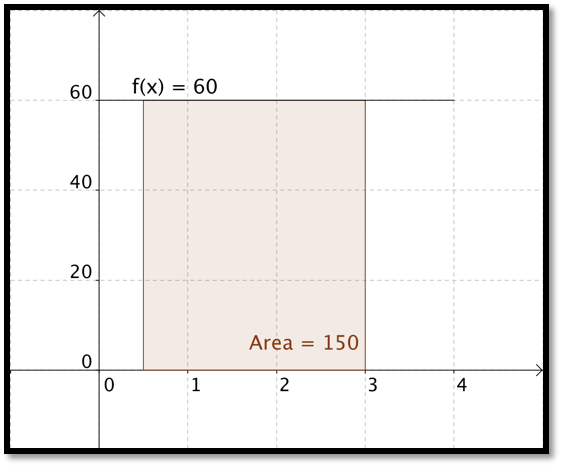### Checkpoint7.1.10.Reading check, Approximating Definite Integrals as Sums.

This question checks your reading comprehension of the material is section 7.1, Approximating Definite Integrals as Sums, of Business Calculus with Excel. Based on your reading, select all statements that are correct. There may be more than one correct answer. The statements may appear in what seems to be a random order.
1. This sections gave a list of formulas of definite integrals of nice functions.
2. We can approximate the area under a curve with a sum of rectangular areas.
3. The text mentioned a memory aid that uses the Greek and Latin alphabets.
4. One of the examples in the section looked at finding the volume of a solid of revolution.
5. For functions in this class it does not matter is our sum uses right or left hand points to form the rectangle in a subinterval.
6. One of the examples in the section looked at finding the present value of a revenue stream.
7. The limit of a Riemann Sum is a Definite Integral.
8. None of the above

### ExercisesExercises: Approximating Definite Integrals as Sums Problems

#### 1.

Let $$f(x) = 4 x + 5\text{.}$$ Estimate the area under $$f(x)$$ on the interval $$0 \le x \lt 7$$ using 100 rectangles and a right hand rule.
Solution.
The Excel commands are: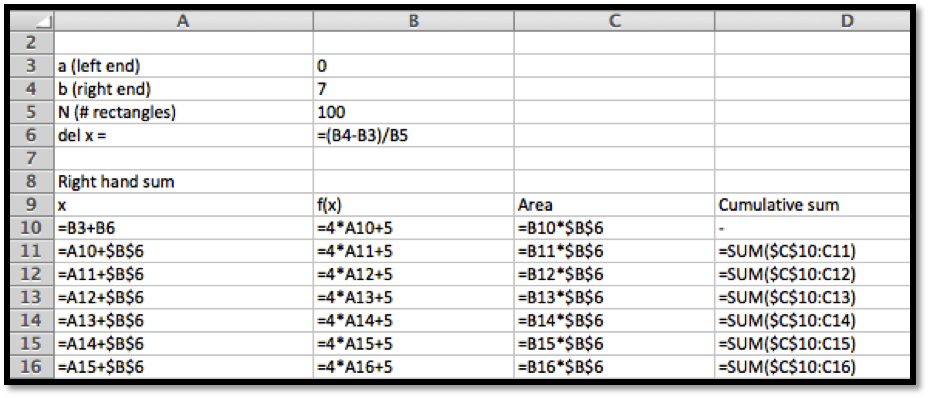The answer is given as follows. Note that in this screen grab the center part of the sidebyside was hidden so that the image is a reasonable size. The area is approximately 133.98.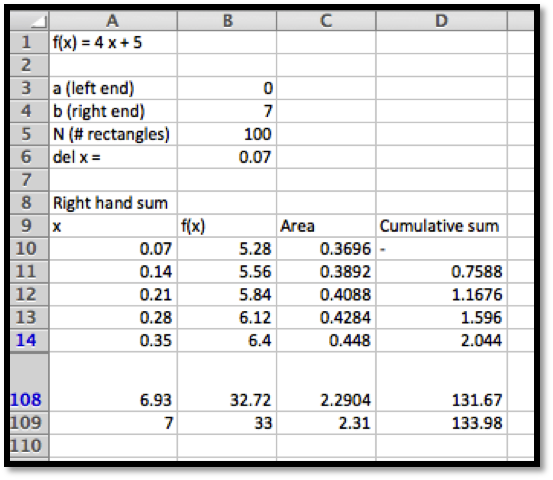#### 2.

Let $$f(x) = 5 - 3 x\text{.}$$ Estimate the area under $$f(x)$$ on the interval $$2 \le x \lt 10$$ using 200 rectangles and a right hand rule.

#### 3.

Let $$f(x) = x^2 + 3 x + 1\text{.}$$ Estimate the area on the interval $$-10\le x \lt -2$$ under $$f(x)$$ using 200 rectangles and a right hand rule.
Solution.
The set-up is very similar to the one in problem 1.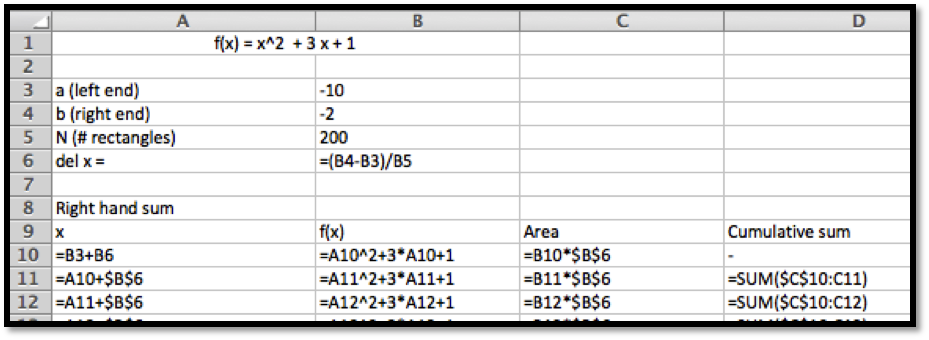The area underneath the curve is approximately 193.228 according to the Excel computation.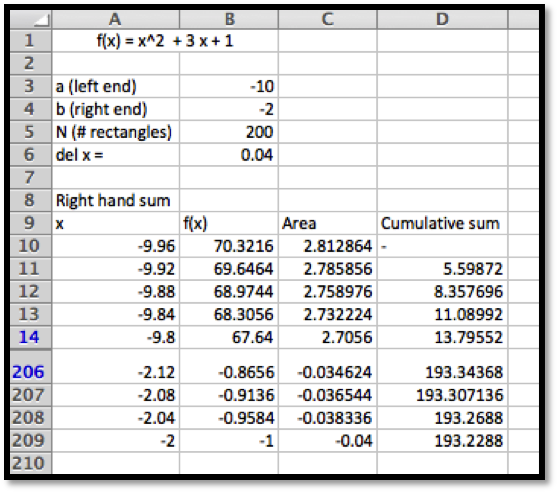#### 4.

Let $$f(x) = -x^2 + 7 x - 10\text{.}$$ Estimate the area below the curve $$y = f(x)$$ and above the x-axis using 100 rectangles and a right hand rule.

#### 5.

Let $$f(x) = 3 \ln(x)\text{.}$$ Estimate the area under $$f(x)$$ on the interval $$1 \le x \lt 10$$ using 50 rectangles and a right hand rule.
Solution.
A set-up similar to the one in problems 1 and 3 gives us an approximation for the area of 42.69.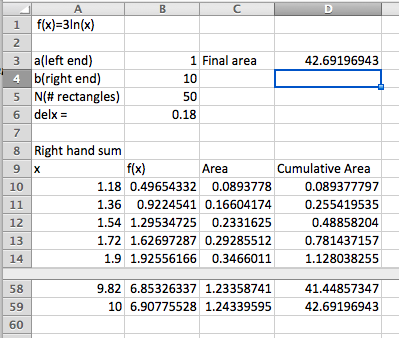#### 6.

Let $$f(x) = x \exp(-0.7 x)\text{.}$$ Estimate the area on the interval $$1 \le x \lt 5$$ under $$f(x)$$ using 100 rectangles and a right hand rule.

#### 7.

Let $$f(x) = (5 x + 3) \exp(-0.7 x)\text{.}$$ Estimate the area under $$f(x)$$ on the interval $$0 \le x \lt 5$$ using 100 rectangles and a right hand rule.
Solution.
The area underneath the curve is approximately 12.92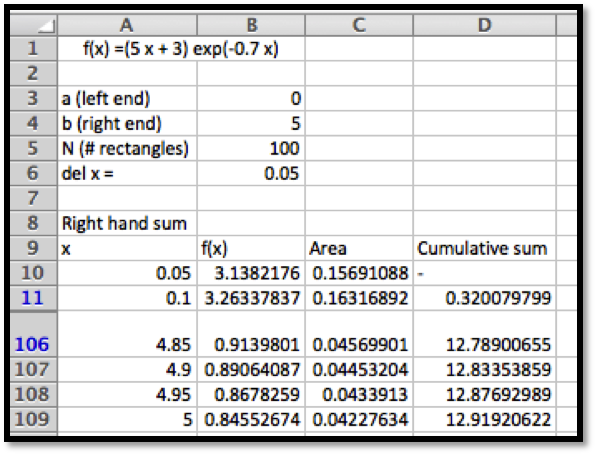#### 8.

Consider the area under the line $$y = 5 x + 7$$ on the interval $$1 \le x \le 5\text{.}$$
1. Using only what you know about areas of rectangles and triangles, find the exact area.
2. Find the approximations to the area using Riemann sums with 50, 100, and 200 intervals.
3. Find the error for each of the three approximations you made.
4. For this case, make an estimate of the error in terms of the number of intervals used.

#### 9.

Consider the area under the line $$y = x^2$$ on the interval $$0 \le x \le 3\text{.}$$ In later sections we will show that the exact area is 9.
1. Find the approximations to the area using Riemann sums with 1, 10, 100, and 1000 intervals.
2. Find the error for each of the four approximations you made.
3. For this case, make an estimate of the error in terms of the number of intervals used.
4. How many intervals would we need for an error of less that $$10^{-6}\text{?}$$
Solution.
1.  N Riemann Sum Error 1 27 18 10 10.395 1.395 100 9.135 0.135 1000 9.0135 0.0135
2. The errors are included in the sidebyside above. Subtract 9 from the approximation found in Excel. Note that there is a bit of a pattern.
3. The larger values of N seem to have an error of about $$\frac{13.5}{N}$$
4. If error $$\lt 10^{-6}\text{.}$$ Then $$\frac{13.5}{N}\lt 10^{-6}\text{.}$$ Then $$\frac{N}{13.5}\gt 1,000,000\text{,}$$ and hence $$N\gt 13,500,000\text{.}$$

#### 10.

You have a natural gas well. You have been told that as gas is extracted and the pressure in the well lessens, the rate of extraction also decreases. The weekly production is $$10000 \exp(-0.01 t)$$ cubic feet per week.
1. Estimate the production in the first year.
2. Estimate the production in the third year.

#### 11.

Sales of your new gadget are estimated at $$500*2^{.08 t}$$ units per month.
1. Estimate the total sales in the first year.
2. Estimate the total sales in the fourth year.
3. Estimate the total sales over the first 5 years.
Solution.
1. Estimate the total sales in the first year.
The total sales would be the sum of the sales each month. This is the same as a right hand sum of the function $$\Sales(t)= 500*2^{.08 t}$$ on the interval $$[0,12]$$ with 12 subdivisions. The Excel commands are as follows (quick fill down to complete the Excel table):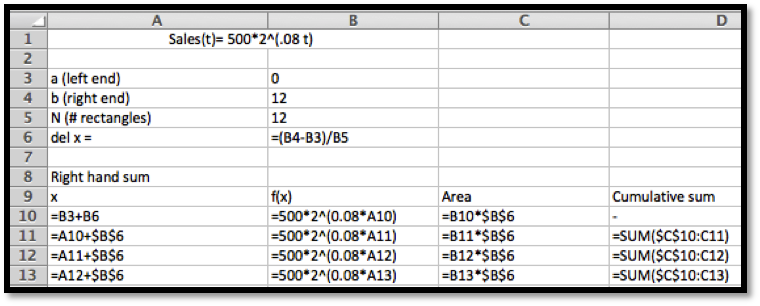Results: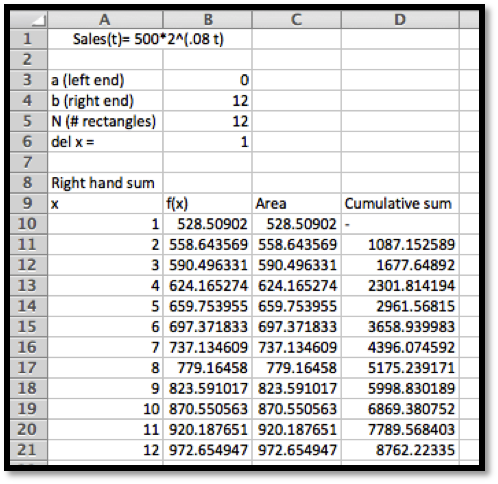So the total sales is $8,762.22 2. Estimate the total sales in the fourth year. We need to adjust the computation so that the sales added correspond to the sales of the fourth year only. This would be from month 36 to 48. We can just adjust the values in the Excel table above!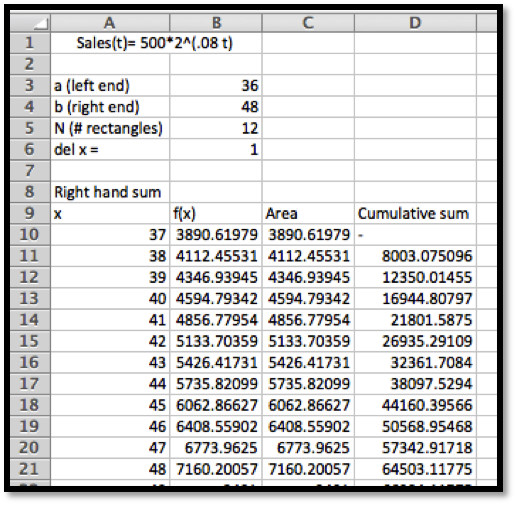So the total sales in the fourth year are$64,503.12
3. Estimate the total sales over the first 5 years.
This will be a much larger range. We will add the sales for the first 5 years. In other words: the first 60 months. Note that this means we want to change $$N$$ to 60 (we want to do the computation for each month). The rows from 13 to 65 have been hidden from views to create a smaller size image for this solution manual: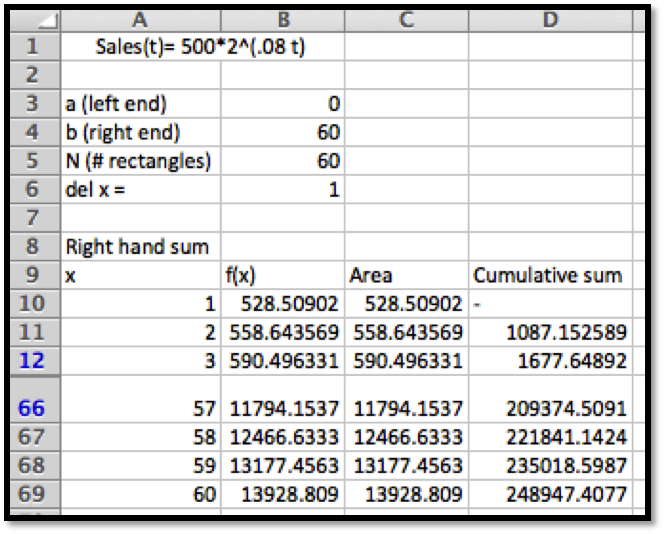The total sales for the first 5 years is \$248,947.41

#### 12.

You run a low cost, high volume widget manufacturing plant. For reports, you write your reports in terms of millions of units. When measured in units of one million widgets and one million dollars, the marginal profit function is $$p(x) = -1 + 10 x - x^2\text{.}$$
1. Find the profit from making 12 million widgets.
2. What quantities have 0 marginal profit?
3. What is the maximum profit to be made manufacturing widgets?
mathstat.slu.edu/~may/ExcelCalculus/external/Examples/Section-7-1-Examples.xlsx